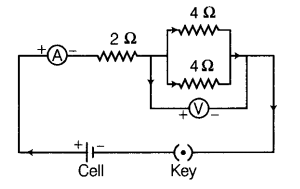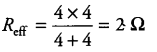# Draw a circuit diagram of an electric circuit containing a cell, a key, an ammeter

Draw a circuit diagram of an electric circuit containing a cell, a key, an ammeter, a resistor of 2 ohms in series with a combination of two resistors (4 ohms each) in parallel and a voltmeter across the parallel combination. Will the potential difference across the 2 ohms resistor be the same as that across the parallel combination of 4 ohms resistors? Give reasons.
Make a rough sketch of the circuit using the material given with proper connection, then solve it.

The circuit is shown as the figure:Effective resistance of combination of two resistors (4 ohms each) in parallel isSince, the resistor of 2 ohms and parallel combination of two 4 ohms resistors are in series, same current will flow through these. Hence, the potential difference across 2 ohms resistor is same as that across combination of two resistors.